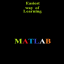MATLAB TUTORIALS - Slunečnice.cz Hlavní navigace

#MATLAB TUTORIALS 1.2

3

Pro hodnocení programu se prosím nejprve

Staženo 0 ×
Zdarma

## Sdílet

This app enables you to learn basic MATLAB which is mandatory before learning any toolbox of MATLAB. This app contains complete notes on following topics:
MATLAB Arrays
MATLAB Functions
MATLAB Script file
MATLAB Loops
MATLAB Two dimensional plots
MATLAB application in calculus
MATLAB Solution of differential equation using MATLAB
This app has a very simple user interface and the content can be easily understood by the user. Programs are also given along with theory to develop algorithm making skill. Wherever and whenever you require any information about MATLAB you are just a click away............

All the best............

1.2

• #### Poslední aktualizace od vývojáře

18. 11. 2017 2017-11-18

0 ×

• Vzdělávání
• #### Podporované jazyky

• Angličtina
Zobrazit více
3

Pro hodnocení programu se prosím nejprve

Staženo
0 ×

TIP: Stahují se vám programy pomalu? Změřte si rychlost svého internetového připojení.